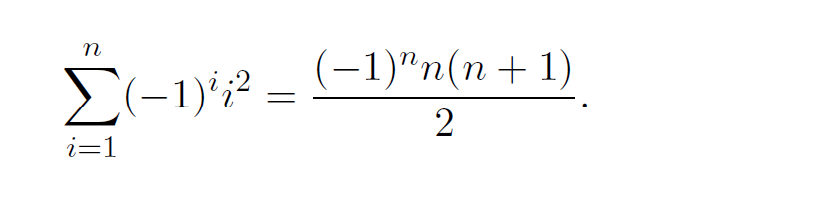# Question Prove by induction that for all positive integers n. Answer must follow the steps below: 1- Clearly state P(n) 2- Basis of induction: Chose an appropriate n0 and prove that P(n0) is true. 3- Induction Step: Prove that P(k) --> P(k+1) is true for all k>= N0 4- Conclusion n Σ(-1): (-1)*n(n+1) 2 1=1XQ7FZZ The Asker · Other Mathematics

Prove by induction that for all positive integers n.1- Clearly state P(n)

2- Basis of induction: Chose an appropriate n0 and prove that P(n0) is true.

3- Induction Step: Prove that P(k) --> P(k+1) is true for all k>= N0

4- Conclusion

Transcribed Image Text: n Σ(-1): (-1)*n(n+1) 2 1=1
More
Transcribed Image Text: n Σ(-1): (-1)*n(n+1) 2 1=1